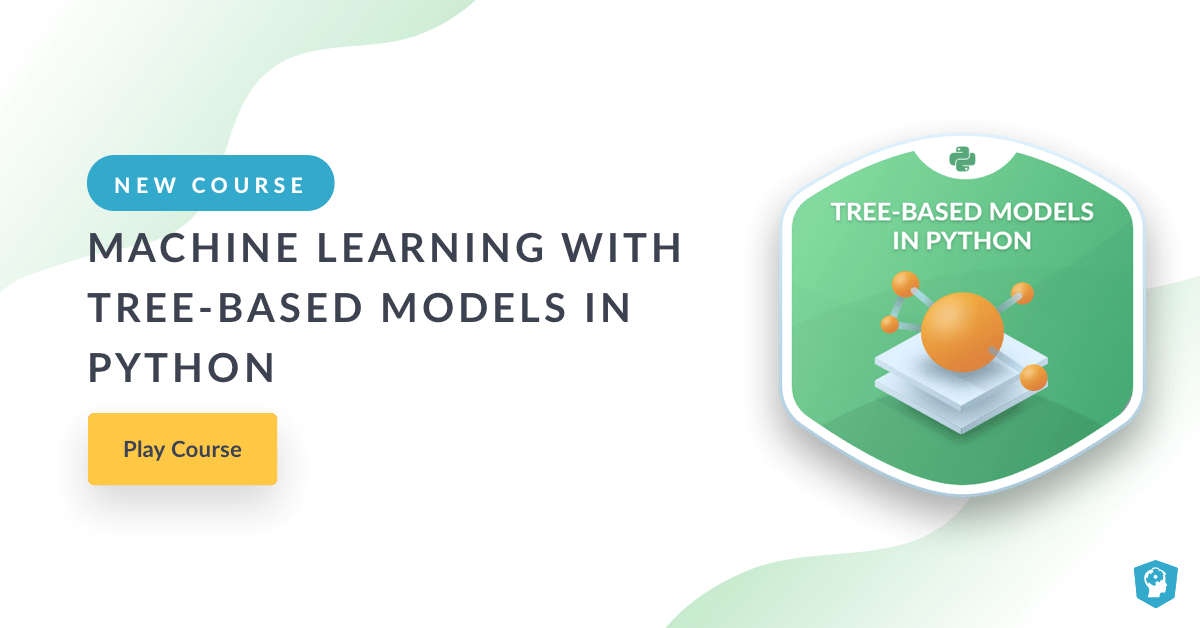# New Course: Machine Learning with Tree-Based Models in Python

Learn about our new Python course. You'll learn how to use Python to train decision trees and tree-based models with the user-friendly scikit-learn machine learning library.
Jul 2018  · 3 min readHere is the course link.

### Course Description

Decision trees are supervised learning models used for problems involving classification and regression. Tree models present a high flexibility that comes at a price: on one hand, trees are able to capture complex non-linear relationships; on the other hand, they are prone to memorizing the noise present in a dataset. By aggregating the predictions of trees that are trained differently, ensemble methods take advantage of the flexibility of trees while reducing their tendency to memorize noise. Ensemble methods are used across a variety of fields and have a proven track record of winning many machine learning competitions. In this course, you'll learn how to use Python to train decision trees and tree-based models with the user-friendly scikit-learn machine learning library. You'll understand the advantages and shortcomings of trees and demonstrate how ensembling can alleviate these shortcomings, all while practicing on real-world datasets. Finally, you'll also understand how to tune the most influential hyperparameters in order to get the most out of your models.

### Chapter 1: Classification and Regression Trees (Free)Classification and Regression Trees (CART) are a set of supervised learning models used for problems involving classification and regression. In this chapter, you'll be introduced to the CART algorithm.

### Chapter 2: The Bias-Variance TradeoffThe bias-variance tradeoff is one of the fundamental concepts in supervised machine learning. In this chapter, you'll understand how to diagnose the problems of overfitting and underfitting. You'll also be introduced to the concept of ensembling where the predictions of several models are aggregated to produce predictions that are more robust.

### Chapter 3: Bagging and Random ForestsBagging is an ensemble method involving training the same algorithm many times using different subsets sampled from the training data. In this chapter, you'll understand how bagging can be used to create a tree ensemble. You'll also learn how the random forests algorithm can lead to further ensemble diversity through randomization at the level of each split in the trees forming the ensemble.

### Chapter 4: BoostingBoosting refers to an ensemble method in which several models are trained sequentially with each model learning from the errors of its predecessors. In this chapter, you'll be introduced to the two boosting methods of AdaBoost and Gradient Boosting.

### Chapter 5: Model TuningThe hyperparameters of a machine learning model are parameters that are not learned from data. They should be set prior to fitting the model to the training set. In this chapter, you'll learn how to tune the hyperparameters of a tree-based model using grid search cross-validation.

### Prerequisites

Supervised Learning with scikit-learn